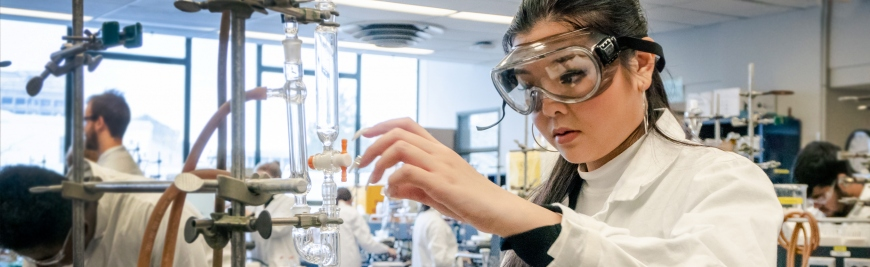## Research & Teaching Faculty# IONIC-CONDUCTIVITY IN THE WATER-RICH REGION OF AQUEOUS 2-BUTOXYETHANOL

 Title IONIC-CONDUCTIVITY IN THE WATER-RICH REGION OF AQUEOUS 2-BUTOXYETHANOL Publication Type Journal Article Year of Publication 1995 Authors Koga, Y, Loo, VJ, Puhacz, KT Journal Canadian Journal of Chemistry-Revue Canadienne De Chimie Volume 73 Pagination 1294-1297 Date Published Aug Type of Article Article ISBN Number 0008-4042 Keywords AQUEOUS 2-BUTOXYETHANOL, BOND NETWORK, HYDROGEN, IONIC CONDUCTIVITIES, MIXING SCHEME, MIXING SCHEMES, MIXTURES, PARTIAL MOLAR ENTHALPIES, SCATTERING, TRANSITION, VOLUMES Abstract Ionic conductivities of HCl, KOH, and KCl were measured in aqueous solutions of 2-butoxyethanol (BE) at 25 degrees C. The quantity, Lambda(j)’ = sigma/x(j), which is almost proportional to the molar conductivity, was extrapolated to the infinite dilution x(j) –> 0. sigma is the conductivity and x(j) is the mole fraction of j(= HCl, KOH, or KCl). The plots of (0) Lambda(j)’, the value of Lambda(j)’ extrapolated to infinite dilution, against x(BE) showed a change in slope at x(BE) = 0.0175. The previous work from this laboratory indicated that the mixing scheme changes qualitatively at the same locus, x(BE) = 0.0175. By mixing scheme we simply mean the way in which BE and H2O molecules mix with each other. Assuming additivity in (0)h(j)’ in terms of constituent ions, those values for H+OH- were calculated. Plots of (0) Lambda(H+OH-)’ thus calculated as a function of x(BE) in the water-rich region, 0 < x(BE) < 0.0175, suggest that the hydrogen bond probability decreases in the bulk of solution, as x(BE) increases. URL ://A1995RZ49200008